﻿ 异质性劳动力流动、房价变动与要素价格扭曲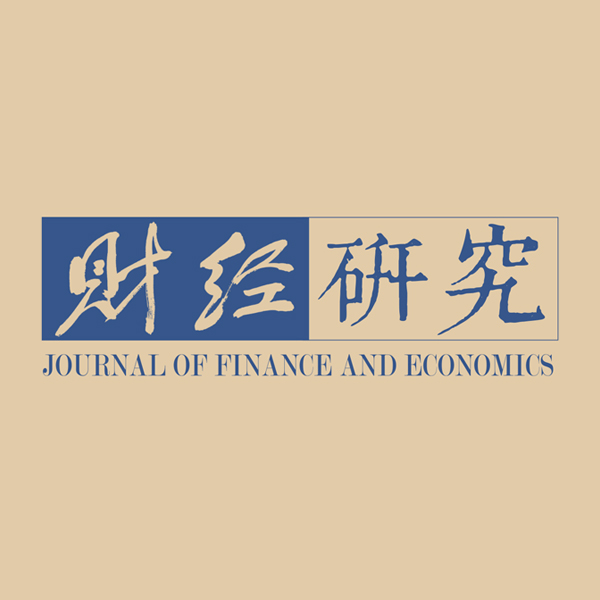《财经研究》
2022第48卷第6期

Heterogeneous Labor Migration, Housing-price Changing and Factor-price Distortion
Xu Shaojun, Sun Wei
Center for Quantitative Economics, Jilin University, Changchun 130012, China
Summary: Although Chinese economy has achieved rapidly growth in short term, the price distortion of land, labor and other factors restricted the realization of high-quality development. How to tame the inflation of housing prices, alleviate factor-price distortion and optimize the factor-allocation among regions has become an urgent problem to be solved. Under this background, this paper attempts to theorize the endogenous mechanism between housing-price formation, heterogeneous labor migration and regional factor-price distortion. By constructing a two-region theoretical model with real estate, this paper studies the interdependence between real sector, services and real estate, and expounds the linkage between factor flowing, factor-price distortion and housing prices. Numerical simulation reveals that labor skill distribution property determines the degree of factor-price distortion, migration scale and housing-price formation. The migration and agglomeration of skilled labor will induce capital and unskilled labor agglomeration, and further lead housing-price rising through the scale effect and crowding out unskilled labor. Besides, industrial interdependence will impede the crowding-out effect on unskilled labor to a certain extent. Finally, it leads to endogenoushouse price formation and labor skill structure changing across regions. The above theoretical findings have been empirically proofed by Chinese provincial data from 2004 to 2019. Skilled labor aggregation leads to negative labor-price distortion and positive capital-price distortion; housing-price rising leads to positive labor-price distortion and negative capital-price distortion. Finally, skilled labor agglomeration has a negative effect on total-factor-price distortion and relative-factor-price distortion. Housing-price rising has a positive effect on total-factor-price distortion and relative-factor-price distortion. The potential contributions of this paper are as follows: Firstly, under the framework of two-region theoretical model, labor with heterogeneous skills is distinguished, which indicates the mechanism of skill structure, factor allocation and factor-price distortion caused by heterogeneous labor migration. Secondly, this paper proves that the induced effect caused by heterogeneous labor migration can offset the crowding-out effect on unskilled labor agglomeration to a certain extent. Thirdly, this paper finds that the skill structure improvement brought by skilled labor agglomeration and its induced effect on capital are the key to housing-price rising. The rise of housing prices has crowded out the unskilled labor of low bargaining power, and induced the substitution of capital for labor, forming an endogenous feedback mechanism of housing-price rising, labor skill structure improving and capital deepening in skill agglomerating areas. These contributions provide a new perspective for understanding the housing bubble formation, and clarify the mechanism of heterogeneous factor allocation and efficiency.
Key words: heterogeneous labor migration    housing-price changing    factor-price distortion

（一）居民偏好

 $U = \max \;\sum\limits_{t = 0}^\infty {{\beta ^t}\left[ {\ln (\frac{{C_{B,t}^\sigma }}{\sigma } + {C_{M,t}}) + \gamma \ln {C_{H,t}}} \right]}$ (1)
 $s.t.\;\;P_{B,t}^d \cdot C_{B,t}^d + P_{M,t}^d \cdot C_{M,t}^d + P_{H,t}^d \cdot \Delta C_{H,t}^d + S_{t + 1}^d = (1 + {r_t})S_t^d + W_t^d\;\;\;\;\;d \in \left( {l,h,j} \right)$ (2)

 $C_{B,t}^d = {(\frac{{{P_{B,t}}}}{{{P_{M,t}}}})^{\frac{1}{{\sigma - 1}}}}$ (3)
 $C_{M,t}^d = \frac{1}{{1 + \gamma }}\frac{{w_t^d}}{{{P_{H,t}}}} + \frac{1}{{\gamma + 1}}\frac{{{P_{H,t}}}}{{{P_{M,t}}}}\bar \omega {_{t-1}^d} - \frac{{\gamma + \sigma }}{{\sigma (\gamma + 1)}}{(\frac{{{P_{B,t}}}}{{{P_{M,t}}}})^{\frac{\sigma }{{\sigma - 1}}}}$ (4)
 $\Delta C_{H,t}^d = \frac{\gamma }{{\gamma + 1}}\frac{{w_t^d}}{{{P_{H,t}}}} - \frac{1}{{\gamma + 1}}\bar \omega {_{t-1}^d -1} + \frac{{\gamma (1 - \sigma )}}{{\sigma (1 + \gamma )}}\frac{{{P_{M,t}}}}{{{P_{H,t}}}}{(\frac{{{P_{B,t}}}}{{{P_{M,t}}}})^{\frac{\sigma }{{\sigma - 1}}}}$ (5)

 ${P_{H,t}} = \frac{{\gamma \cdot {{\bar w}_t} \cdot {O_t}}}{{(1 + \gamma )Y_{Ht}^s + {{\bar \varpi }_t} \cdot {O_t}}}\left[ {1 + \frac{{(1 - \sigma ){P_{M,t}} \cdot {O_t}}}{\sigma }{{(\frac{{{P_{B,t}}}}{{{P_{M,t}}}})}^{\frac{\sigma }{{\sigma - 1}}}}} \right]$ (6)

（二）服务品厂商

 ${C_{B,t}} = {(\int_0^q {c_{i,t}^\rho di} )^{\frac{1}{\rho }}}$ (7)

 ${P_{B,t}} = {(\int_0^q {p_{i,t}^{\frac{\rho }{{\rho - 1}}}di} )^{\frac{{\rho - 1}}{\rho }}}$ (8)

 ${x_{i,t}} = {O_t}{(\int_0^q {p_{i,t}^{\frac{\rho }{{\rho - 1}}}di} )^{\frac{{\rho - 1}}{{\rho (\sigma - 1)}}}} \cdot P_{M,t}^{\frac{1}{{1 - \sigma }}} \cdot q_t^{ - 1}$ (9)

 ${x_t} = {O_t}q_t^{\frac{{\rho - 1}}{{\rho (\sigma - 1)}}}{p_t}^{\frac{1}{{\sigma - 1}}}$ (10)

 $W_t^J = {(\frac{{{O_t}}}{{{J_t}}})^{1{\text{ - }}\sigma }}q_t^{\frac{{1 - \rho }}{\rho }}{(\frac{{2\sigma - 1}}{{{a_T}\sigma }})^\sigma }$ (11)

 ${x_t} = \frac{{W_t^JF}}{{{p_t} - {a_T}W_t^J}}\;$ (12)

 ${\pi _{i,t}} = {p_{i,t}}{x_{i,t}} - W_{i,t}^J({F_i} + {a_T}{x_{i,t}})$ (13)
 $s.t.\;\;\;{x_t} = {O_t}q_t^{\frac{{\rho - 1}}{{\rho (\sigma - 1)}}}{p_t}^{\frac{1}{{\sigma - 1}}}$ (14)

 ${p_t} = \frac{{{a_T} \cdot \sigma \cdot W_t^J}}{{2\sigma - 1}}$ (15)

 ${J_t} = (F + {a_T}{x_t}) = F + \frac{{2\sigma - 1}}{{1 - \sigma }}F = \frac{\sigma }{{1 - \sigma }}F$ (16)

 ${q_t} = \frac{{\overline J }}{{{J_i}}} = \frac{{\overline J (1 - \sigma )}}{{\sigma F}}$ (17)

（三）工业品厂商

 $M_t^s = A_t^s \cdot K_{s,t}^{{a_s}} \cdot H_{s,t}^{{b_s}} \cdot L_{s,t}^{1 - {a_s} - {b_s}}$ (18)

 $w_{s,t}^d = {{W_{s,t}^d} \mathord{\left/ {\vphantom {{W_{s,t}^d} {\left( {P_{M,t}^s \cdot P_{B,t}^s \cdot P_{G,t}^s} \right)}}} \right. } {\left( {P_{M,t}^s \cdot P_{B,t}^s \cdot P_{G,t}^s} \right)}}\;\;\;\;\;d \in (h,l,j)$ (19)
 $r_t^s = {a_s}K_{s,t}^{{a_s} - 1}H_{s,t}^{{b_s}}L_{s,t}^{1 - {a_s} - {b_s}}$ (20)

（四）房地产厂商

 ${Y_{H,t}} = z{G_t}$ (21)

 ${H_t} \cdot \Delta C_{H,t}^h + {L_t} \cdot \Delta C_{H,t}^l + {J_t} \cdot \Delta C_{H,t}^j = z \cdot {G_{H,t}}$ (22)

（五）异质性要素流动

 $H_{t + 1}^s = (1 + h)H_t^s + {\psi _h} \cdot H_t^n \cdot {{(w_{s,t}^h - w_{n,t}^h)} \mathord{\left/ {\vphantom {{(w_{s,t}^h - w_{n,t}^h)} {w_{s,t}^h}}} \right. } {w_{s,t}^h}}$ (23)
 $L_{t + 1}^s = (1 + n)L_t^s + {\psi _l} \cdot L_t^n \cdot {{(w_{s,t}^l - w_{n,t}^l)} \mathord{\left/ {\vphantom {{(w_{s,t}^l - w_{n,t}^l)} {w_{s,t}^l}}} \right. } {w_{s,t}^l}}$ (24)
 $J_{t + 1}^s = (1 + n)J_t^s + {\psi _j} \cdot J_t^n \cdot {{(w_{s,t}^j - w_{n,t}^j)} \mathord{\left/ {\vphantom {{(w_{s,t}^j - w_{n,t}^j)} {w_{s,t}^j}}} \right. } {w_{s,t}^j}}$ (25)

 $K_{t + 1}^s = (1 + {g_k})K_t^s + {\psi _k} \cdot K_t^n \cdot {{({r_{s,t}} - {r_{n,t}})} \mathord{\left/ {\vphantom {{({r_{s,t}} - {r_{n,t}})} {{r_{s,t}}}}} \right. } {{r_{s,t}}}}$ (26)

（一）参数校准

 参数及取值 经济含义 理论依据 as=0.43 流入地的资本产出弹性 投入产出表的要素收入份额占GDP比重；孙巍和徐邵军（2021） bs=0.24 流入地的技能型劳动力产出弹性 （1-as-bs）=0.35 流入地的非技能型劳动力产出弹性 an=0.41 流出地的资本产出弹性 bn=0.21 流出地的技能型劳动力产出弹性 （1-an-bn）=0.32 流出地的非技能型劳动力产出弹性 Fs=Fn=300 地区服务业的固定资产使用总额 Mori（2012）；何雄浪和毕佳丽（2014） aTs=aTn=0.3 产品转化效率 ρ=4 异质性服务品替代参数 gs=0.12 流入地区的房地产年平均增长率 国信房地产信息网 gn=0.17 流出地区的房地产年平均增长率 β=0.99 主观贴现因子 李尚骜和龚六堂（2012） gk=0.15 资本的稳态增长率（储蓄率） σ=0.55 风险规避系数 主观贴现率测算 γ=0.3 住房偏好参数 郭念枝和村濑英彰（2018） ψh=0.05 技能型劳动力的流动敏感系数 误差最小化原则测算 ψj=ψl=0.03 普通劳动力、非技能型劳动力的流动敏感系数 ψk=0.05 资本的流动敏感系数 h=0.02 技能型劳动力的平均增长率 《劳动与就业统计年鉴》 n=0.01 非技能型劳动力平均增长率

（二）数值模拟图 1 地区异质性劳动力流动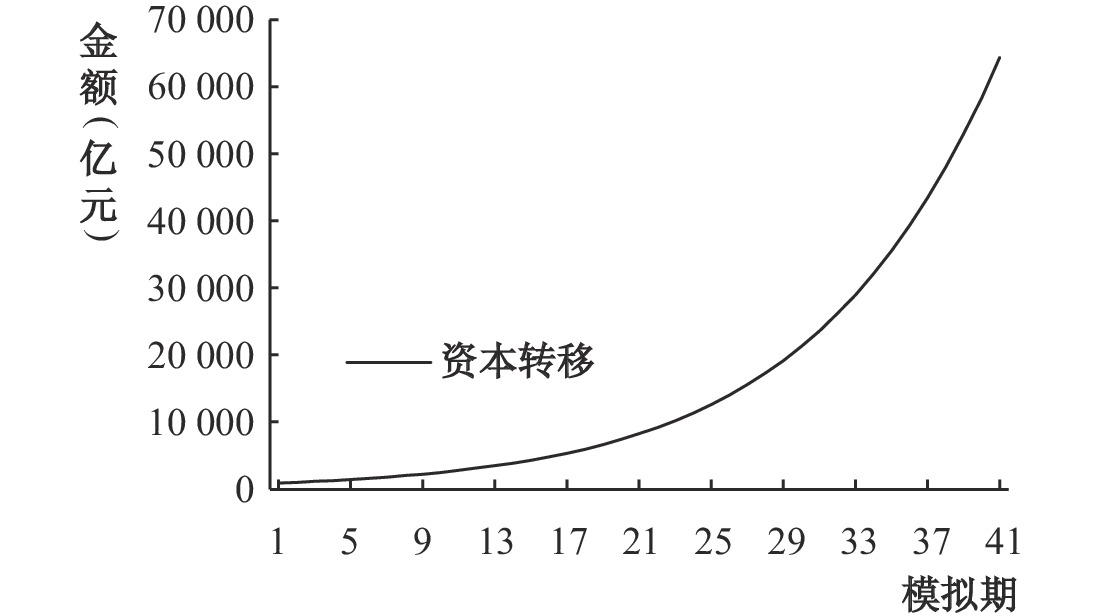图 2 地区资本流动图 3 地区住房价格分化图 4 流入地技能型劳动力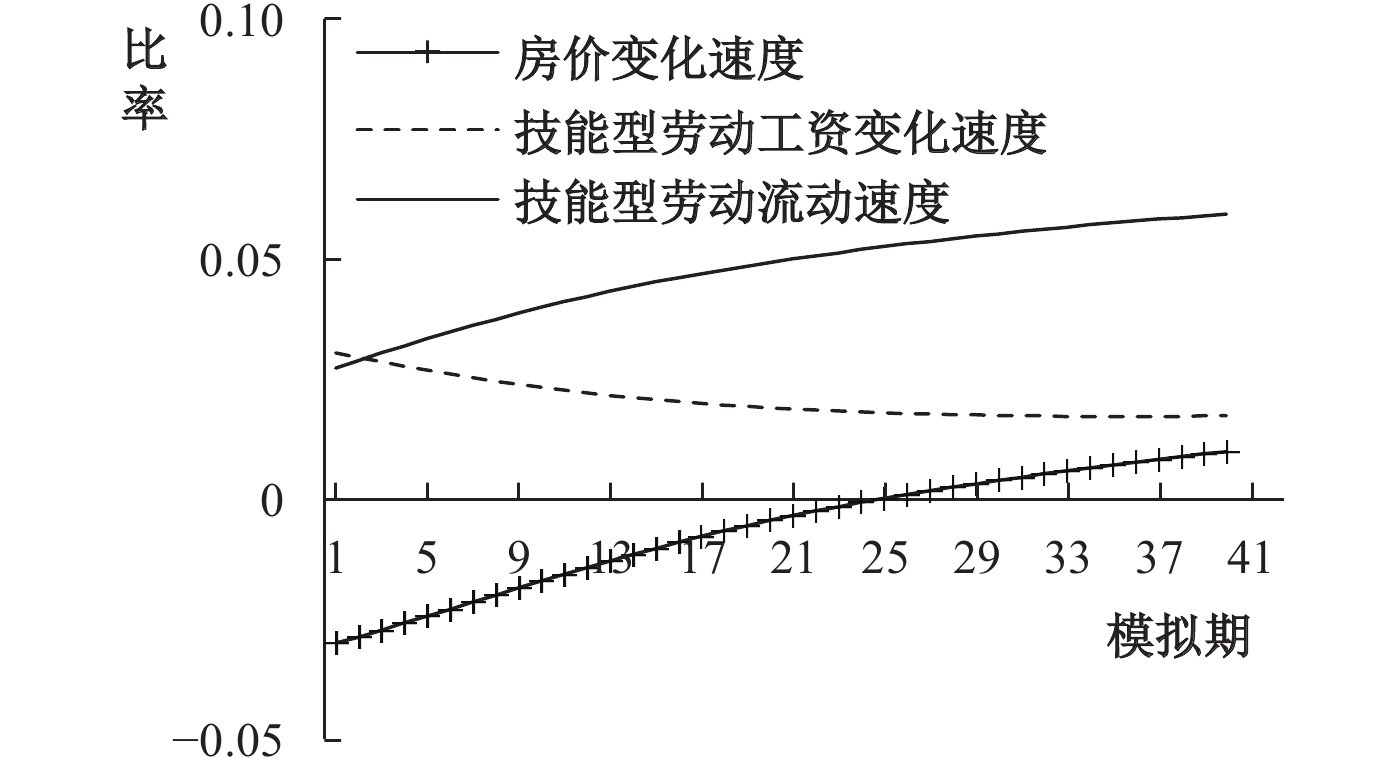图 5 流出地技能型劳动力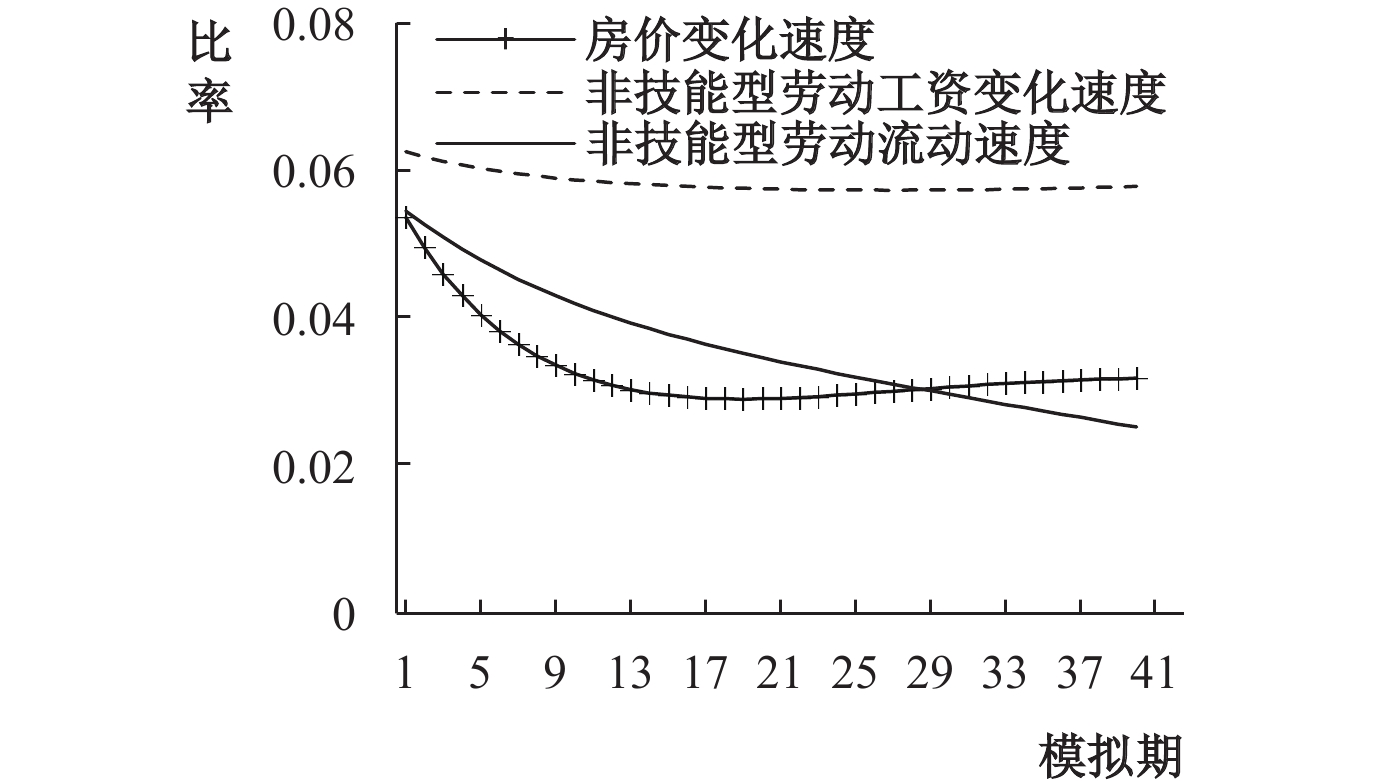图 6 流入地非技能型劳动力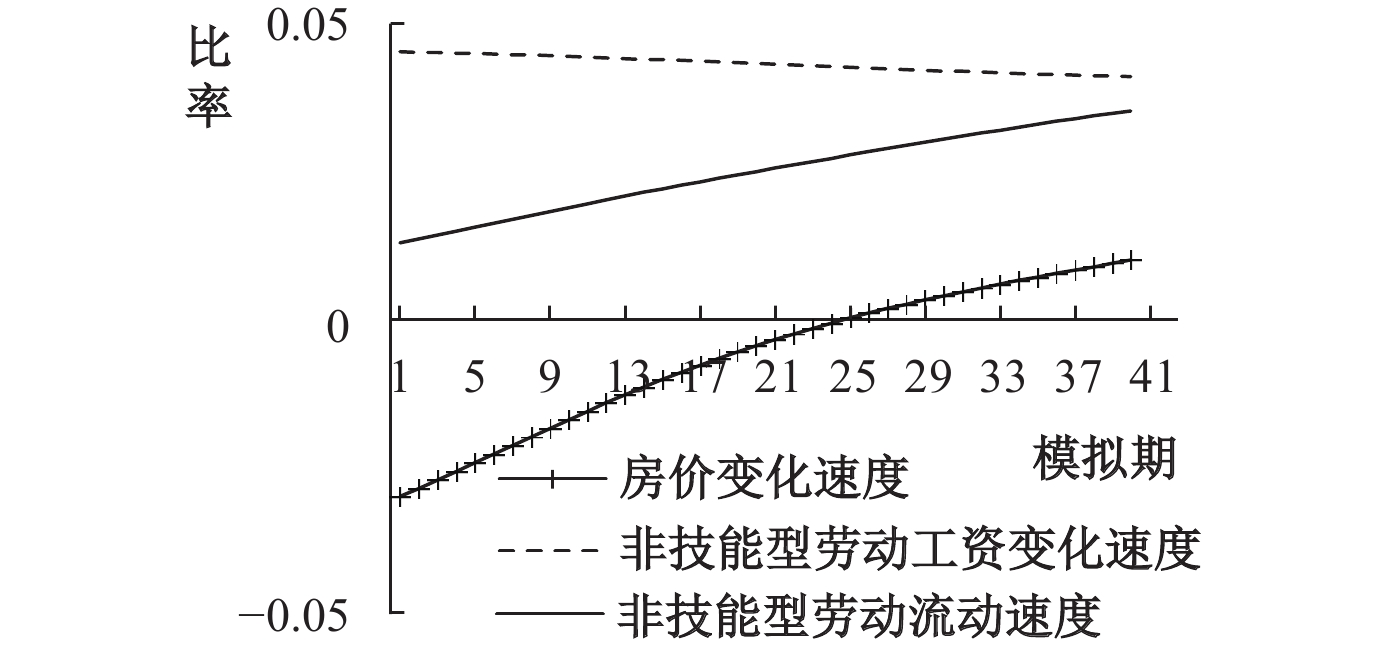图 7 流出地非技能型劳动力图 8 流入地普通劳动力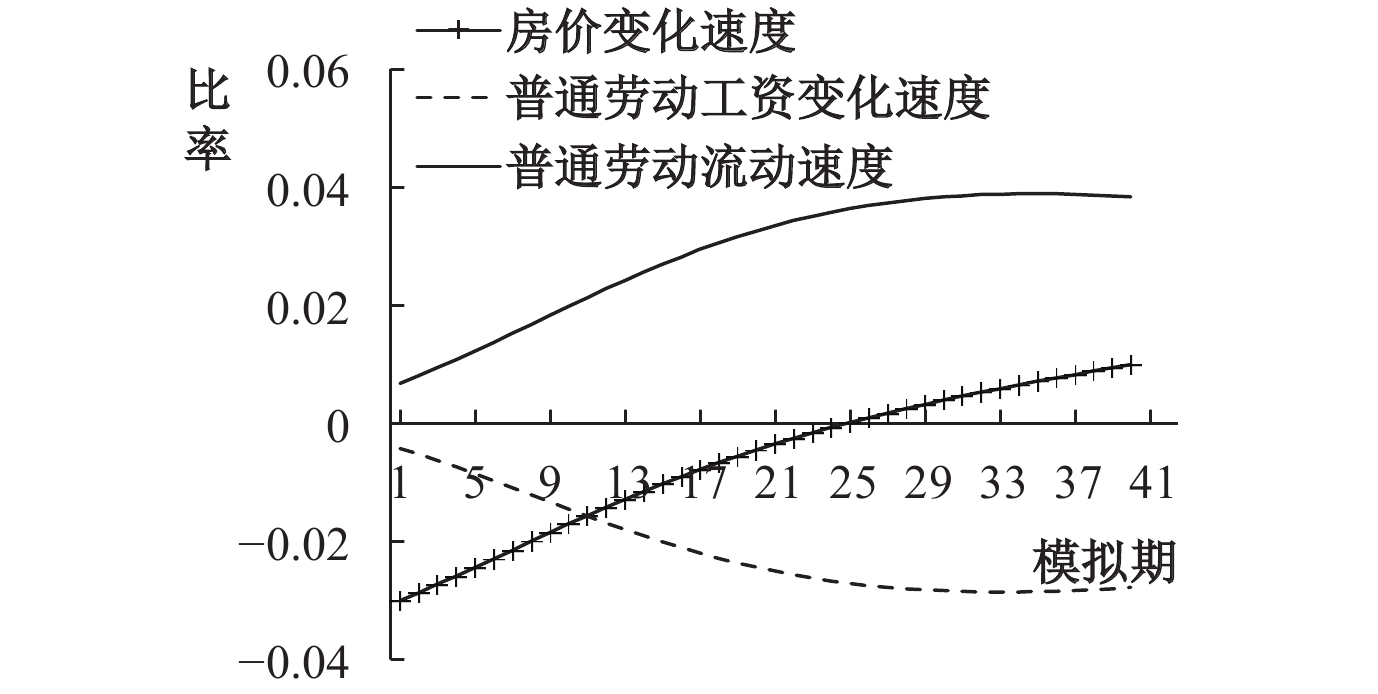图 9 流出地普通劳动力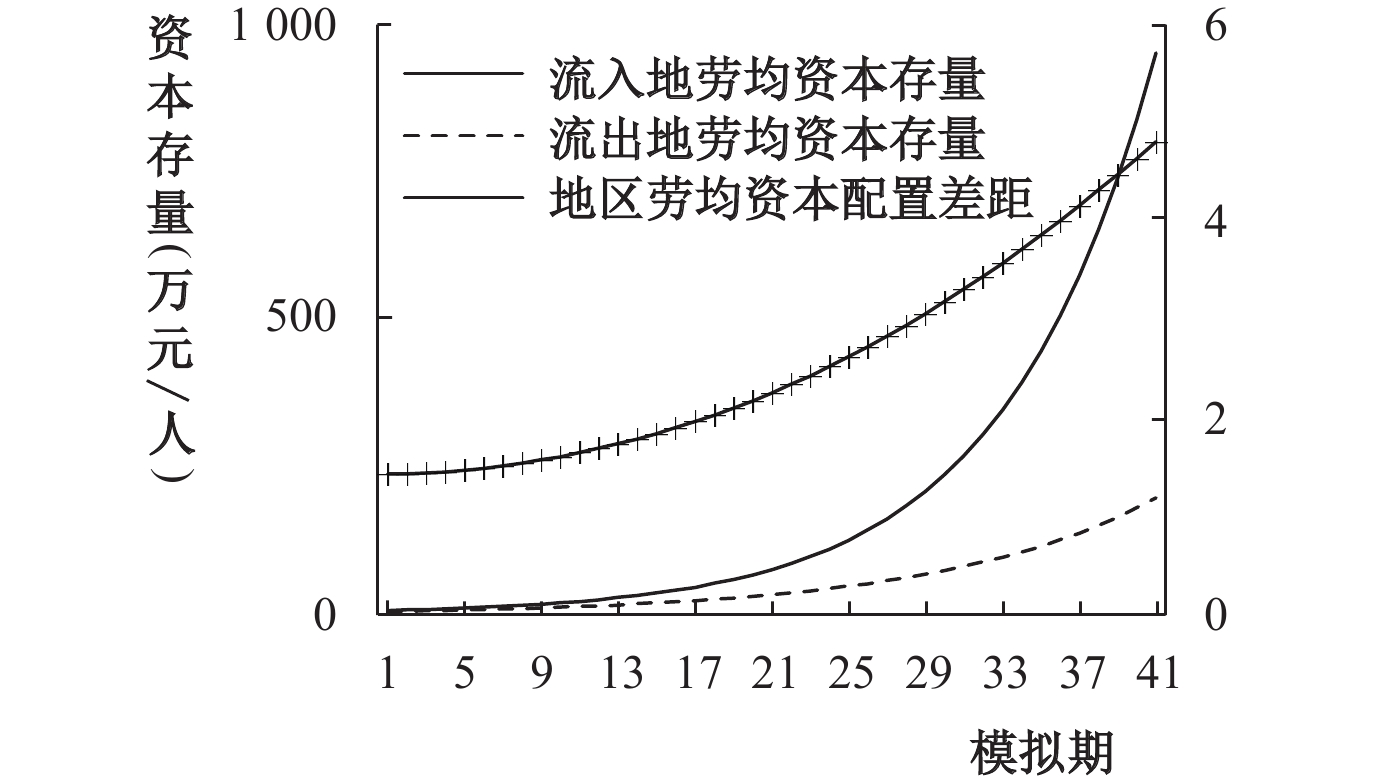图 10 劳均资本存量 注：地区间劳均资本配置差距为比值，将其作次坐标轴显示。本文将所有涉及比值的变量均以次坐标轴反映，下图同。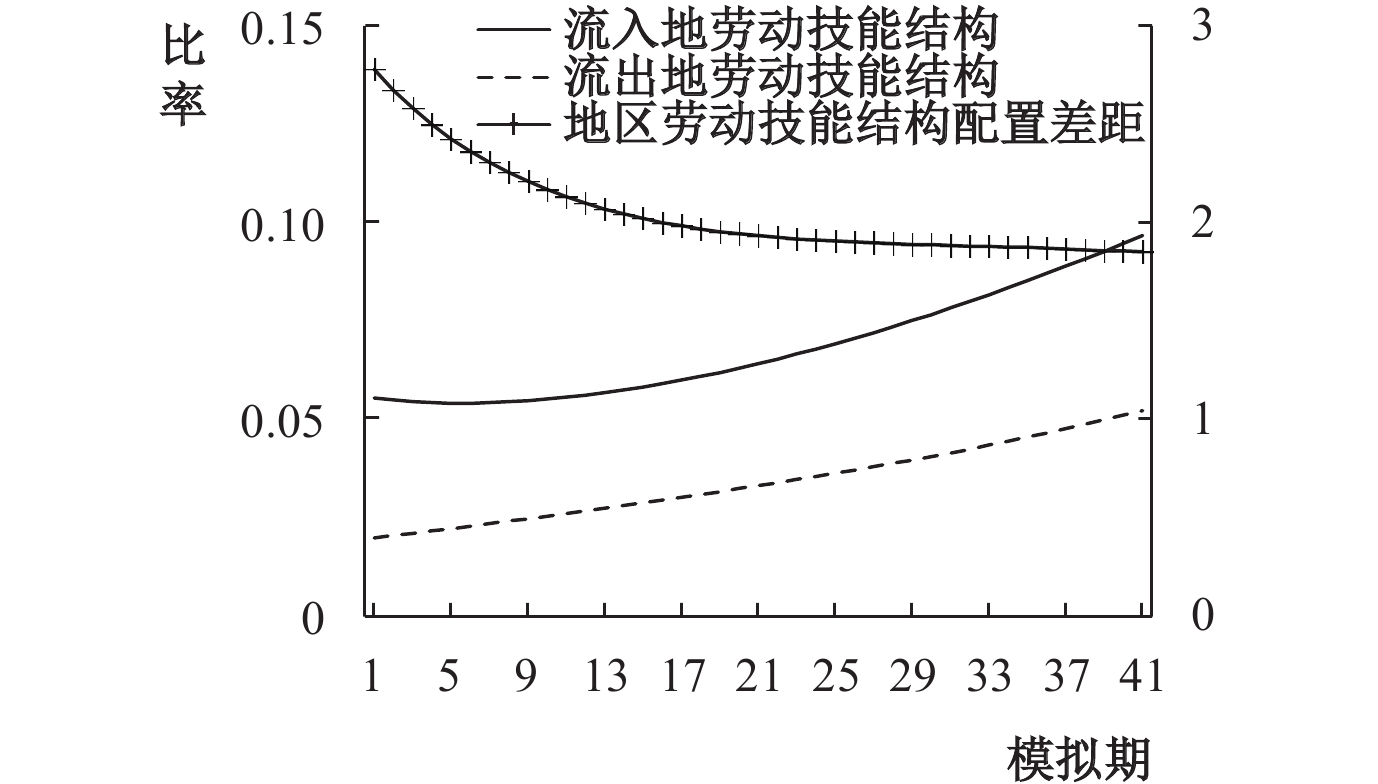图 11 劳动力技能结构

（一）变量构造及数据选取

 $total\_dis{t_{i,t}} = distK_{i,t}^{\frac{\alpha }{{\alpha + \beta }}}distL_{i,t}^{\frac{\beta }{{\alpha + \beta }}}$ (27)
 $relative\_dis{t_{i,t}} = {{dist{L_{i,t}}} \mathord{\left/ {\vphantom {{dist{L_{i,t}}} {dist{K_{i,t}}}}} \right. } {dist{K_{i,t}}}}$ (28)

 变量名称 符号 测度方式 要素相对价格扭曲 relative_dist relative_distit=distLit/distKit 要素整体价格扭曲 total_dist $total\_dis{t_{i,t}} = distK_{it}^{{\alpha \mathord{\left/ {\vphantom {\alpha {\alpha + \beta }}} \right. } {\alpha + \beta }}} \cdot distL_{it}^{{\beta \mathord{\left/ {\vphantom {\beta {\alpha + \beta }}} \right. } {\alpha + \beta }}}$ 房价 ph 地区商品房平均售价的对数 技能型劳动力 skill 地区本科及以上学历劳动力的对数 非技能型劳动力 lab 地区劳动总量的对数 流动比率 mig （常住人口−户籍人口）/户籍人口 资本 cap 永续盘存法计算资本存量的对数

（二）空间溢出效应分析

 $I = \frac{{\sum\limits_{i = 1}^n {\sum\limits_{j = 1}^n {{w_{i,j}}\left( {{x_i} - \overline x } \right)\left( {{x_j} - \overline x } \right)} } }}{{{S^2}\sum\limits_{i = 1}^n {\sum\limits_{j = 1}^n {{w_{i,j}}} } }}$ (29)

 时间 2004 2005 2006 2007 2008 2009 2010 2011 相对扭曲 0.229 (1.585) 0.337***(2.876) 0.464***(6.324) 0.480***(5.168) 0.440***(3.795) 0.340***(3.001) 0.305**(2.239) 0.309**(2.267) 整体扭曲 0.252**(2.081) 0.265***(2.854) 0.285***(4.523) 0.264***(3.857) 0.374***(3.763) 0.365***(3.048) 0.353**(2.012) 0.447**(2.064) 时间 2012 2013 2014 2015 2016 2017 2018 2019 相对扭曲 0.271*(1.643) 0.244 (1.377) 0.264**(2.088) 0.271**(2.181) 0.242*(1.701) 0.198**(2.133) 0.215*(1.801) 0.207*(1.795) 整体扭曲 0.428**(2.063) 0.442*(1.759) 0.421**(2.136) 0.395***(2.703) 0.342**(2.050) 0.414 (1.638) 0.403**(2.132) 0.417**(2.112) 注：小括号内为z值。***、**和*分别表示在 1%、5% 和 10% 的显著性水平下显著。

（三）要素扭曲的实证检验

 ${z_{i,t}} = \tau {z_{i,t - 1}} + \rho {w'_i}{z_t} + {x'_{i,t}}\beta + {d'_i}{X_t}\delta + {\mu _i} + {\gamma _t} + {\varepsilon _{i,t}}$ (30)

 要素整体价格扭曲 要素相对价格扭曲 经济距离阵 空间权重阵 替代变量 经济距离阵 空间权重阵 替代变量 skill 0.172**(0.0815) 0.0721**(0.0344) 0.406***(0.0764) 0.0129***(0.00389) 0.0696*(0.0392) 0.106**(0.0499) ph −0.0560**(0.0252) −0.562***(0.0889) −0.0831**(0.0359) −0.164***(0.0495) −0.204***(0.0618) −0.108***(0.0342) mig 1.266**(0.642) 1.027***(0.391) 1.133***(0.325) −0.520**(0.214) −0.608***(0.213) −0.513**(0.208) cap −0.346***(0.113) −0.397***(0.0787) −0.323***(0.0725) 0.0224 (0.0309) 0.0313 (0.0340) 0.0407 (0.0265) lab 0.665***(0.250) 0.173 (0.122) 0.585***(0.0972) −0.189*(0.102) −0.271***(0.0758) −0.0436 (0.140) W×skill −24.18 (14.93) −1.173 (10.47) −3.745 (2.856) −7.018***(1.169) −3.389 (6.748) −10.28***(1.350) W×ph 17.59*(10.40) −8.029 (11.05) −13.63 (9.525) 14.01***(4.854) 10.10**(4.522) 10.40**(4.682) W×mig 61.33 (91.80) 28.46 (27.57) 37.04 (23.96) 25.72 (28.59) 42.90*(24.17) 33.80 (27.86) W×cap 4.007 (11.14) 3.254 (6.371) −0.210 (4.956) 7.789***(3.007) 6.626**(3.084) 8.114***(2.841) W×lab −11.93 (20.21) 6.002 (11.36) 19.63*(10.20) −12.92**(6.486) −24.57***(5.783) −4.291 (7.008) $\rho$ −2.071**(1.0497) −0.366**(0.163) −1.922***(0.576) −0.658***(0.182) −1.068**(0.481) −1.317**(0.601) sigma2 0.253***(0.0125) 0.296***(0.0197) 0.291***(0.0190) 0.0413***(0.00631) 0.0425***(0.00266) 0.0409***(0.00613) 时间固定效应 控制 控制 控制 控制 控制 控制 空间固定效应 控制 控制 控制 控制 控制 控制 N 480 480 480 480 480 480 R2 0.4783 0.4278 0.4357 0.3895 0.3710 0.3953 注：小括号内数值为经稳健标准误调整后的t值。***、**和*分别表示在 1%、5% 和 10% 的显著性水平下显著，下表同。

① 除了要素流动方程外，流入地和流出地的其他所有方程形式完全一致。本文所建立的模型，除需特殊备注说明，所有方程只写出流入地相应方程的表达形式，各变量略去表示地区的下标。

② 同地区厂商数量可能不同，这也是模型求解的关键结论之一。

③ 由于篇幅限制，更为详细的参数校准过程可向作者索取。

④ 数值模拟过程中计算的“工资”指的是相应劳动的边际产出。

⑤ 限于篇幅，分析表格省略，读者若是感兴趣可向作者索取。

⑥ 本文首先进行LM检验，结果表明SEM模型、SAR模型均适用。其次，LR检验拒绝了原假设，说明SDM模型不会简化为SEM模型或SAR模型。再次，Hausman检验结果支持固定效应。最后，联合显著性检验拒绝了原假设，说明选用时间和个体双重效应的SDM模型较为合适。

  陈斌开, 金箫, 欧阳涤非. 住房价格、资源错配与中国工业企业生产率[J]. 世界经济, 2015(4): 77–98.  陈宇峰, 贵斌威, 陈启清. 技术偏向与中国劳动收入份额的再考察[J]. 经济研究, 2013(6): 113–126.  高波, 陈健, 邹琳华. 区域房价差异、劳动力流动与产业升级[J]. 经济研究, 2012(1): 66–79.  郭念枝, 村濑英彰. 劳动力成本上升、资产泡沫与中国经济波动[J]. 经济学(季刊), 2018(3): 949–968.  郭卫军, 黄繁华. 高技术产业与生产性服务业协同集聚如何影响经济增长质量?[J]. 产业经济研究, 2020(6): 128–142.  韩立彬, 陆铭. 供需错配: 解开中国房价分化之谜[J]. 世界经济, 2018(10): 126–149.  何雄浪, 毕佳丽. 技术溢出、要素流动与经济地理均衡[J]. 中央财经大学学报, 2014(4): 80–86.  李尚骜, 龚六堂. 非一致性偏好、内生偏好结构与经济结构变迁[J]. 经济研究, 2012(7): 35–47.  李言. 中国生产要素价格扭曲的变迁: 2000-2016年[J]. 经济学动态, 2020(1): 34–51.  李勇刚, 罗海艳. 土地资源错配阻碍了产业结构升级吗?——来自中国35个大中城市的经验证据[J]. 财经研究, 2017(9): 110–121.  刘贯春, 张晓云, 邓光耀. 要素重置、经济增长与区域非平衡发展[J]. 数量经济技术经济研究, 2017(7): 35–56.  陆铭, 张航, 梁文泉. 偏向中西部的土地供应如何推升了东部的工资[J]. 中国社会科学, 2015(5): 59–83.  罗知, 张川川. 信贷扩张、房地产投资与制造业部门的资源配置效率[J]. 金融研究, 2015(7): 60–75.  蒲艳萍, 顾冉. 劳动力工资扭曲如何影响企业创新[J]. 中国工业经济, 2019(7): 137–154.  宋弘, 吴茂华. 高房价是否导致了区域高技能人力资本流出?[J]. 金融研究, 2020(3): 77–95.  孙巍, 徐邵军. 技术进步路径转换、异质性劳动力流动与地区经济差距演化[J]. 经济评论, 2021(5): 65–85.  王宁, 史晋川. 中国要素价格扭曲程度的测度[J]. 数量经济技术经济研究, 2015(9): 149–161.  王小鲁, 胡李鹏, 樊纲. 中国分省份市场化指数报告(2021)[M]. 北京: 社会科学文献出版社, 2021.  汪勇, 李雪松. 外生冲击、房地产价格与企业投资[J]. 财经研究, 2019(3): 60–72.  王竹泉, 王苑琢, 王舒慧. 中国实体经济资金效率与财务风险真实水平透析——金融服务实体经济效率和水平不高的症结何在?[J]. 管理世界, 2019(2): 58–73. DOI:10.3969/j.issn.1002-5502.2019.02.005  吴海民. 资产价格波动、通货膨胀与产业“空心化”——基于我国沿海地区民营工业面板数据的实证研究[J]. 中国工业经济, 2012(1): 46–56.  谢申祥, 陆毅, 蔡熙乾. 开放经济体系中劳动者的工资议价能力[J]. 中国社会科学, 2019(5): 40–59.  徐朝阳, 林毅夫. 发展战略与经济增长[J]. 中国社会科学, 2010(3): 94–108.  张巍, 许家云, 杨竺松. 房价、工资与资源配置效率——基于微观家庭数据的实证分析[J]. 金融研究, 2018(8): 69–84.  周颖刚, 蒙莉娜, 卢琪. 高房价挤出了谁?——基于中国流动人口的微观视角[J]. 经济研究, 2019(9): 106–122.  Hsieh C T, Klenow P J. Misallocation and manufacturing TFP in China and India[J]. The Quarterly Journal of Economics, 2009, 124(4): 1403–1448. DOI:10.1162/qjec.2009.124.4.1403  Levinsohn J, Petrin A. Estimating production functions using inputs to control for unobservables[J]. The Review of Economic Studies, 2003, 70(2): 317–341. DOI:10.1111/1467-937X.00246  Miao J J, Wang P F. Sectoral bubbles, misallocation, and endogenous growth[J]. Journal of Mathematical Economics, 2014, 53: 153–163. DOI:10.1016/j.jmateco.2013.12.003  Mori T. Increasing returns in transportation and the formation of hubs[J]. Journal of Economic Geography, 2012, 12(4): 877–897. DOI:10.1093/jeg/lbr028  Rong Z, Wang W C, Gong Q. Housing price appreciation, investment opportunity, and firm innovation: evidence from China[J]. Journal of Housing Economics, 2016, 33: 34–58. DOI:10.1016/j.jhe.2016.04.002